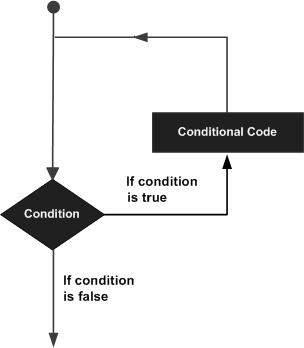Python Loop - How to use Loops in Python | w3Schools

The code in Python is executed in a sequential manner line by line. But sometimes a certain block of code has to be executed several times. This can be done either by defining a function or by defining a loop based on the required condition. Many control structures are available in Python language that can be used for such applications.

###### Also Read: Python Basic Operators

A loop enables the execution of single or multiple statements multiple times. The diagram below shows the working of a loop statement:Following types of loops can be used in python language:

1.      While loop: A while loop statement executes a block of statements repeatedly till the defined condition is True. The condition is tested at every iteration and then the block of code is executed. For example:

count = 0

while (count < 9):

print ‘The count is:’, count

count = count + 1

print “Good bye!”

The above code gives the below runs till the value count is less than 9 and gives the below output:

The count is: 0

The count is: 1

The count is: 2

The count is: 3

The count is: 4

The count is: 5

The count is: 6

The count is: 7

The count is: 8

Good bye!

2.      For loop: A bock of code is executed multiple times after the expression list is evaluated. For example:

fruits = [‘banana’, ‘apple’,’mango’]

for index in range(len(fruits)):

print ‘Current fruit :’, fruits[index]

print “Good bye!”

The above set of code gives the below output:

Current fruit: banana

Current fruit: apple

Current fruit: mango

Good bye!

3.      Nested loop: One loop can also be called within another loop in Python language. The indentation is used to decide which statements belong to which loop. For example:

i = 2

while(i < 10):

j = 2

while(j <= (i/j)):

if not(i%j): break

j = j + 1

if (j > i/j) : print i, ” is prime”

i = i + 1

The above code gives output:

2 is prime

3 is prime

5 is prime

7 is prime

Loop Control Statements

Loop control statements help control the loop execution and their sequence can be changed from the standard flow. Below control statements are supported by Python:

1.      Break statement: It terminates the loop depending on the condition.

var = 7

while var > 0:

print ‘Current variable value :’, var

var = var -1

if var == 5:

break

The output of the above code is:

Current variable value : 7

Current variable value : 6

2.      Continue statement: This statement helps in skipping the remaining loop depending on the condition defined in the loop. For example:

for a letter in ‘Python’:

if letter == ‘h’:

continue

print ‘Current Letter :’, letter

The output of the code is:

Current Letter : P

Current Letter: y

Current Letter: t

Current Letter: o

Current Letter: n

3.      Pass statement: This is used when a statement is required syntactically and the remaining command in the block shouldn’t be executed. For example:

for a letter in ‘Python’:

if letter == ‘h’:

pass

print ‘This is pass block’

print ‘Current Letter :’, letter

print ‘The End!’

The output of the above code is:

Current Letter : P

Current Letter: y

Current Letter: t

This is pass block

Current Letter: h

Current Letter: o

Current Letter: n

The End

Conclusion

The above tutorial gives details about the different loops that can be used in Python. Start using these loops in your code and make it more efficient and fast.